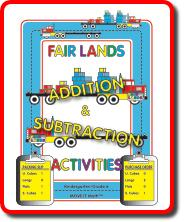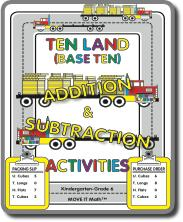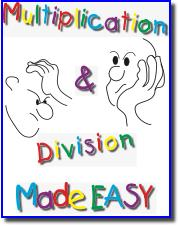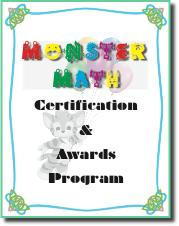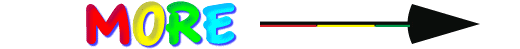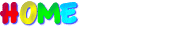Monster Math Certification & Awards Program Add any ten 10-digit numbers   Subtract any two 10-digit numbers   Multiply any size number by any two digits)   Divide any size number by a single digit)Multiplication & Division Made EASY Napier's Bones Lattice Multiplication Stair Step Multiplication Division Worksheets Chunk It Division Super Chunk It Division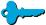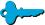MONSTER MATH Addition Subtraction 1-column Addition 2-column Addition Mini-monster Addition Monster Addition Subtraction Facts Mini-monster Subtraction Monster Subtraction Mixed Addition and SubtractionTen Land: Addition & Subtraction Activities in Base 10 Hands-on activities for modeling addition and subtraction in base 10.Fair Lands™: Importing Exporting Activities for Teaching Addition & Subtraction Developmentally appropriate hands-on activities for adding and subtracting in a place value numeration system.